Courses

# Operation on Matrices Mathematics Notes | EduRev

## Algebra for IIT JAM Mathematics

Created by: Veda Institute

## Mathematics : Operation on Matrices Mathematics Notes | EduRev

The document Operation on Matrices Mathematics Notes | EduRev is a part of the Mathematics Course Algebra for IIT JAM Mathematics.
All you need of Mathematics at this link: Mathematics

One of the basic operations that can be performed on matrices is the addition operation. Just as we add two or more integers, two or more matrices can also be added in a similar fashion. This is known as Addition of Matrices. Let’s learn about it in more detail.

Matrix addition is the operation of adding two or matrices by adding the corresponding entry of each matrix together.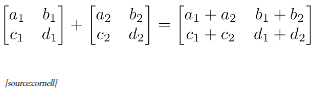The most important rule to know is that when adding two or more matrices, first make sure the matrices have the same dimensions. In order words, you can add a 2 x 3 with a 2 x 3 or a 2 x 2 with a 2 x 2. However, you cannot add a 3 x 2 with a 2 x 3 or a 2 x 2 with a 3 x 3. For example, the addition of two given matrices with dimension 2 × 2,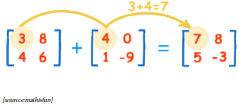1. Commutative Law
If A = [aij], B = [bij] are matrices of the same order, say m × n, then A + B = B + A.

2. Associative Law
For any three matrices A = [aij], B = [bij], C = [cij] of the same order, say m × n, (A + B) + C = A + (B + C).

Let A = [aij] be an m × n matrix and O be an m × n zero matrix, then A + O = O + A = A. In other words, O is the additive identity for matrix addition.

Let A = [aij]m×n be any matrix, then we have another matrix as – A = [–aij]m×n such that A + (–A) = (–A) + A= O. So – A is the additive inverse of A or negative of A.

Solved Examples on Addition of Matrices
Question: Assume X, Y, Z, W and P are matrices of order 2 × n, 3 × k, 2 × p, n × 3, and p × k respectively. The restriction on n, k and p so that PY + WY will be defined are:

1. k = 3, p = n
2. k is arbitrary, p = 2
3. p is arbitrary, k = 3
4. k = 2, p = 3

Solution: In this, the order of P = p × k, Order of W = n × 3, Order of Y = 3 × k. Thus, the order of PY = p×k, when k = 3. And the order of WY = p × k, where p = n Thus option (A) is correct.
Question: If the sum of the matrices [x, x, y], [y, y, z] and [z, 0, 0] is the matrix [10, 5, 5], then what is the value of y?

1. -5
2. 0
3. 5
4. 10

Solution: [x, x, y] + [y, y, z] + [z, 0, 0] = [10, 5, 5]. Therefore x + y + z = 10, x +y = 5, y + z = 5 replacing x +y = 5 in x + y + z = 10. We have, z = 5, Also y + z = 5, Therefore y = 5 – z = 0. Thus option B is correct.

Scalar Multiplication of Matrices
Just as two or more real numbers or two or more matrices can be multiplied, did you know that it is possible to multiply a real number with a matrix or vice-versa? Multiplication of matrices generally falls into two categories, Scalar Matrix Multiplication, in which a single real number is multiplied with every other element of the matrix and Vector Matrix Multiplication wherein an entire matrix is multiplied by another one. In this topic, we will learn about the scalar multiplication of a matrix.

What is Scalar Multiplication of Matrices?
Let’s understand the method with an example. Fatima has doubled the production at a factory A in all categories. Previously quantities (in standard units) produced by factory A were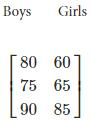Revised quantities produced by factory A are as given below: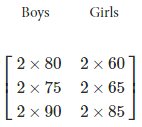his can be represented in the matrix form as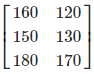We observe that the new matrix is obtained by multiplying each element of the previous matrix by 2. In general, we may define multiplication of a matrix by a scalar as follows:

If A = [aij]m × n is a matrix and k is a scalar, then kA is another matrix which is obtained by multiplying each element of A by the scalar k. In other words, kA = k [aij]m×n = [k (aij)]m×n, that is, (i, j)th element of kA is kaij for all possible values of i and j.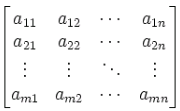Scalars and Scalar Multiplication
When we work with matrices, we refer to real numbers as scalars. The term scalar multiplication refers to the product of a real number and a matrix. In scalar multiplication, each entry in the matrix is multiplied by the given scalar. For example, given that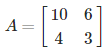let’s find 2A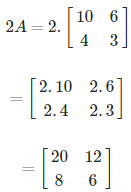Properties of Scalar Multiplication of a Matrix

If A = [aij] and B = [bij] be two matrices of the same order, say m × n, and k and l are scalars, then

• k(A + B) = kA + kB,
(k + l)A = kA + lA
• k (A + B) = k ([aij] + [bij])
= k[aij + bij] = [k(aij + bij)] = [(kaij) + (kbij)]
= [kaij] + [kbij] = k[aij] + k[bij] = kA + kB
• (k + l)A = (k + l)[aij]
= [(k + l)aij] + [kaij] + [laij] = k[aij] + l[aij] = kA + lA

Solved Examples For You
Question: For a matrix A : α(βA) = ? ( Where α and β are real numbers)

1. (αβ)A
2. β(aA)
3. α2A
4. β2A

Solution: Option A or Option B. α and β both are simply the scalars.
Question: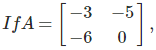A + B = 2I, Find B.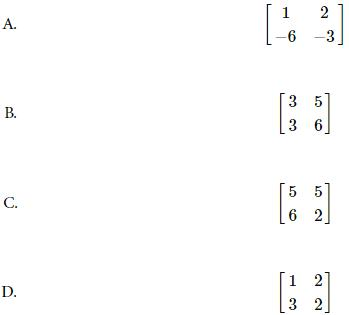Solution: A + B = 2I. Therefore,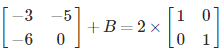Or,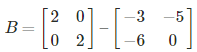So,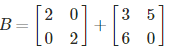Or,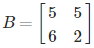Multiplication of Matrices
Just as two or more real numbers can be multiplied, it is possible to multiply two or more matrices too. Multiplication of matrices generally falls into two categories, Scalar Matrix Multiplication, in which a single number is multiplied with every other element of the matrix and Vector Matrix Multiplication wherein an entire matrix is multiplied by another one.

Definition
The product of two matrices A and B is defined if the number of columns of A is equal to the number of rows of B. Let A = [aij] be an m × n matrix and B = [bjk] be an n × p matrix. Then the product of the matrices A and B is the matrix C of order m × p. To get the (i, k)th element c of the matrix C, we take the ith row of A and kth column of B, multiply them element-wise and take the sum of all these products.

In other words, if A = [aij]m×n , B = [bjk]n×p , then the ith row of A = [ai1 ai2 …ain ] and the kth column of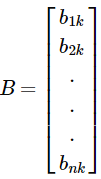then, c = ai1b1k + ai2b2k + ai3b3k + … + ainbnk = ∑aijbjk. The matrix C = Σ[cik]m×p is the product of A and B.

When Do We Do Multiplication?
When the number of columns of the 1st matrix must equal the number of rows of the 2nd matrix. In other words, To multiply an m×n matrix by an n×p matrix, the ns must be the same, and the result is an m×p matrix.

(m×n) × (n×p) → m×p

Matrix Multiplication
Whenever we multiply a matrix by another one we need to find out the “dot product” of rows of the first matrix and columns of the second. Now, what does that mean? Let us see an example below: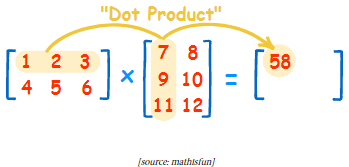The dot product is where we multiply matching members, then sum them up:

(1, 2, 3) . (7, 9, 11) = 1×7 + 2×9 + 3×11 = 58

We match the 1st members (1 and 7), multiply them, likewise for the 2nd members (2 and 9) and the 3rd members (3 and 11), and finally sum them up.

If we multiply a 2×3 matrix with a 3×1 matrix, the product matrix is 2×1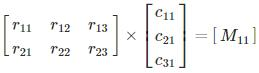Here is how we get M11 and M12 in the product.
M11 = r11× t11  +  r12× t21  +   r13×t31

M12 = r21× t11  +  r22× t21  +  r23×t31

Properties of Matrix Multiplication

1) Associative Law
The assosiative law for any three matrices A, B and C, we have(AB) C = A (BC), whenever both sides of the equality are defined. Example: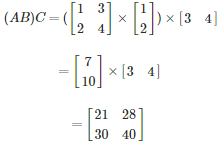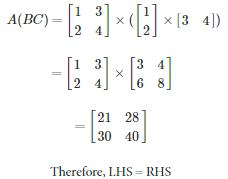2) Distributive Law
The distributive law for three matrices A, B and C.

1. A (B + C) =AB + AC
2. (A + B)  C = AC + BC, whenever both sides of equality are defined.

Example: (i)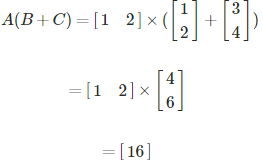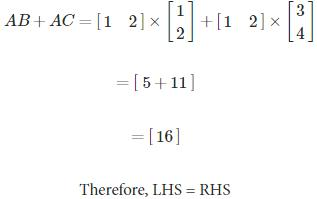Similarly, (ii) can also be proved.

3) Existence of Multiplicative Identity

The existence of multiplicative identity for every square matrix A, there exists an identity matrix of same order such that   IA = AI = A. Example: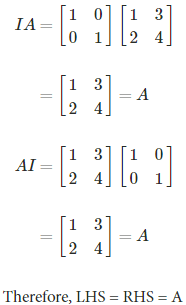Solved Examples For You

Question: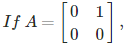I is the unit matrix of order 2 and a,b are arbitrary constants then (aI + bA)2 is equal to

1. a2I + abA
2. a2I + 2abA
3. I2a + b2A
4. None of these

Solution: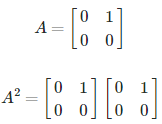⇒ A2 = 0

Now, consider (aI + bA)2 = (aI + bA) (aI + bA)
=a2I + abIA + baAI + b2A2
=a2I + 2abA (∵A2 = O, ab = ba for  real  numbers)

Therefore, the answer is option B

Transpose of a Matrix
What if two matrices have the same set of elements? Will they be necessarily considered equal? Certainly not! This is because their order may be different. Transpose is a matrix formed by swapping the rows into columns and vice-versa. Sounds interesting right? Let’s learn about it in further more detail.

Definition
The new matrix obtained by interchanging the rows and columns of the original matrix is called as the transpose of the matrix. If A = [aij] be an m × n matrix, then the matrix obtained by interchanging the rows and columns of A would be the transpose of A. of It is denoted by A′or (AT). In other words, if A = [aij]mxn ,thenA′ = [aji]nxm . For example,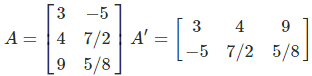Properties

1) Transpose of Transpose of a Matrix

The transpose of the transpose of a matrix is the matrix itself: (AT)T = A. For example,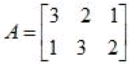Verify that (AT)T = A. It is determined as shown below: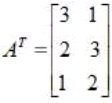Therefore,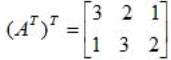2) Transpose of a Scalar Multiple
The transpose of a matrix times a scalar (k) is equal to the constant times the transpose of the matrix: (kA)T = kAT For example,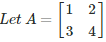and k = 2. Verify that (kA)T = kAT
Solution: LHS = (kA)T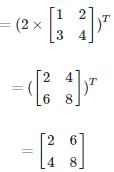RHS = kAT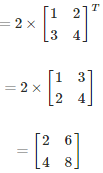Therefore LHS = RHS. Hence, (kA)T = kAT

3) Transpose of a Sum
The transpose of the sum of two matrices is equivalent to the sum of their transposes: (A + B)T = AT + BT. For example: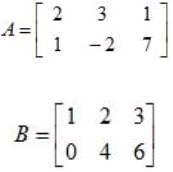verify that (A ± B)T = AT ± BT.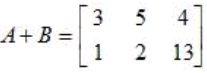Therefore,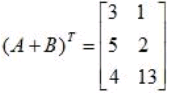The transpose matrices for A and B are given as below: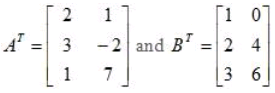Therefore,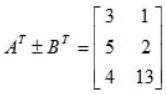Hence (A ± B)T = AT ± BT.

4) Transpose of a Product
The transpose of the product of two matrices is equivalent to the product of their transposes in reversed order: (AB)T = BT AT . The same is true for the product of multiple matrices: (ABC)T = CTBTAT. For example,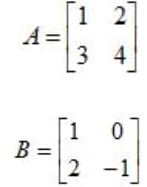verify that (AB)T = BT AT.

Solution: The product of A and B is: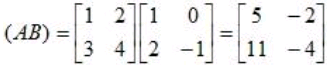If we take the transpose of A and B separately and multiply A with B, then we have: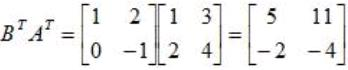Hence (AB)T = BT AT .

Learn the Types of Matrix here.

Solved Examples For You
Question: If matrix A is a circulant matrix whose elements of first row are a, b, c all > 0 such that abc = 1 and ATA = I, then a3 + b3+ c3 equals

1. 0
2. 3
3. 1
4. 4

Solution: Given, A is a circulant matrix of elements a, b, c and abc = 1. So,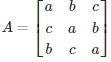So, det A = a(a2 – bc) – b(ac-b2) + c(c2 – ab) = a3 + b3 + c3 – 3 and ATA = I
|ATA| = |I| = 1
|A| |AT| = 1
|A|2 = 1

Therefore, |A| = ±1. So, det A = ± 1. After substituting the value in the det A = a3 + b3 + c3 – 3abc, we get, a3 + b3 + c3 = 4 or  a3 + b3 + c3 = -2. Therefore, answer is option D.

Offer running on EduRev: Apply code STAYHOME200 to get INR 200 off on our premium plan EduRev Infinity!

58 docs

,

,

,

,

,

,

,

,

,

,

,

,

,

,

,

,

,

,

,

,

,

;